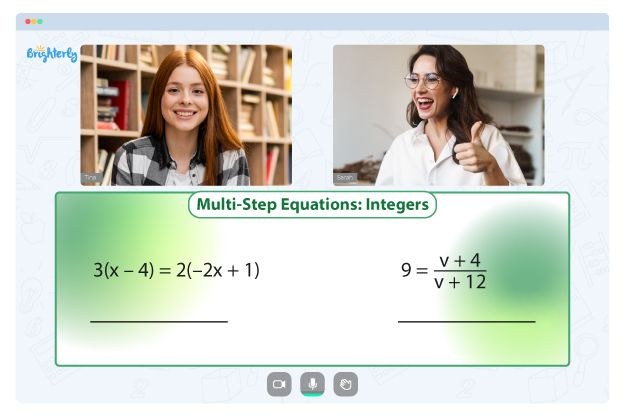# Exponents Worksheets

Exponents mean the expression of large numbers in power. For example, if you want to identify the multiples of a number, you can do it by raising it to the power of that number. For example, 10×10 is 100, but you can say “ten raised to the power of 2” to get the same answer.

Another way to define exponents is to say that it indicates the number of times a number figure can be multiplied by the base. There are many ways to define and explore exponents to help kids understand the concept better.

However, you also need to ensure that students are getting enough study and practice time to master the topic, which is why you need exponents worksheets. With an exponent worksheet, kids can learn all about exponents from the comfort of their homes.

## Benefits of exponents worksheets

There are so many benefits of using the exponents worksheet as teaching materials for kids, and here are some of these benefits:

### Worksheets simplify the learning process

The first time kids learn about multiplication is usually when they have to bring one number together two or multiple times to get an answer. However, as children progress in studying math, they will engage in more complex math processes, which means they no longer have the luxury of time to solve multiples in that manner.

The principle of exponents allows to raise a base to the power of its multiplier to determine the answer to a problem. The use of exponents worksheets pdf to study at home allows kids to understand the idea of exponents more straightforwardly due to repeated practice.

### Worksheets give kids an opportunity to practice

After leaving their class, a kid only has to work with their textbooks, classwork, and sometimes, the assignments the teacher sets. If you want to give your kids more options to practice with, the use of the exponents practice worksheet will cut your work in half.

These worksheets come with over 100 practice test questions, some of which kids do not explore in class because they need to meet the curriculum first. With consistent practice, kids get better at exponents, and teachers no longer have to spend so much time repeating the concept in class for students.

### Worksheets help kids see how they can represent numbers in different ways

As kids grow, they learn that numbers can be represented in many different ways. With exponent worksheets PDF, kids can learn all the different ways to represent numbers.

Math for Kids

Is Your Child Struggling With Math?
1:1 Online Math TutoringFor example, a kid knows that to get 100 from the multiples of 10, they have to say 10x10x10x10x10x10x10x10x10x10. However, when learners look at the worksheet, they know they can now write “ten raised to the power of 2” to get 100. They learn that this principle works for other numbers as well.

If you were worried about creating an extensive worksheet for your kids that encapsulates all the lessons on exponents, you do not need to. On math websites, there are numerous exponents worksheets with answers that you can download to set tests and assignments for your students. In addition, you can print an exponent worksheet PDF off the internet for free. Finally, you can always find an exponents worksheet PDF with fun pictures and colorful designs that your kids would love.### Exponent Worksheet PDF

Exponent Worksheet PDF### Exponents Worksheets PDF

Exponents Worksheets PDF### Exponents Practice Worksheet PDF

Exponents Practice Worksheet### Exponent Worksheets PDF

Exponent Worksheets PDF

Need help with Equations?• Does your child need extra help with understanding of equations?
• Start studying with an online tutor.

Is your child finding it hard to master equations lessons? An online tutor could be of assistance.